# Adding equations to a model

You add equations to your model using the Math view’s equation table. The Concept equation solver solves both geometric and non-geometric equations. As a result, you can add functional properties to your models. You can mix and match dimensions and variables in equations. New variables included in your equations are automatically added to the Math view’s parameter table.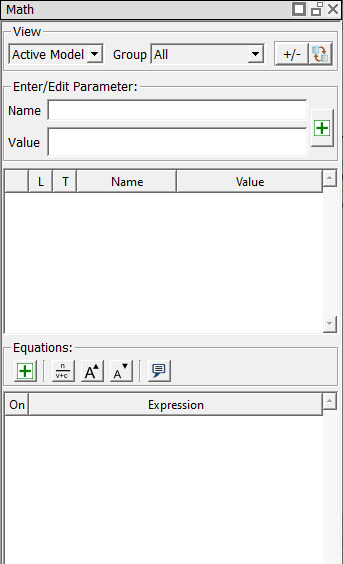In this lesson, we will use equations to compute the maximum fiber stress (normal stress) in a simple rectangular beam. We will assume that the beam is a simply supported beam with a point load (P) at the center.

In step 1 of this lesson, we will draw the cross section of the beam. In step 2, we will create a new math group to contain our equations, and then add the equations to the model.

### Step 1: Draw the beam

1. If needed, start a new file by clicking the Create a new model tool from the main toolbar or selecting New from the File menu.
2. Using the Rectangle tooldraw a small rectangle by clicking two points in the sketch area that define the upper left and lower right diagonal points of the rectangle. Don't worry about exact values of the dimensions yet.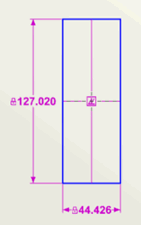Observe that the dimensions for the rectangle also appear in the parameter table (the upper portion of the Math view).Next, we will identify the primary datum surface of the part by placing a fixed point and Line At Angle constraint on one of the lines. We will not place datums in this simple example. For information about datums, see Enventive Concept Help.
3. Add a Fixed Point and Line At Angle constraint to the rectangle in one step by selecting the Fixed Point constraint tool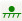and then clicking on the right line of the rectangle. The line at angle constraint is also added to the parameter table, because its value is modifiable.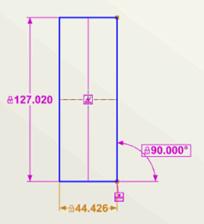4. Observe that the DOF (degrees of freedom) display in the upper right of the Concept window reads 0, indicating the model is fully constrained. The display also shows that our model has one Fixed Point and one Line At Angle constraint.5. In the Parameter table, click anywhere in the row for the Line At Angle dimension. The name and value are shown in the Enter/Edit Parameter area at the top of the Math View. In the Name field, change the dimension's name to LAA. Press Enter to accept the change.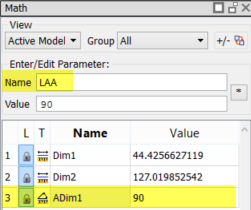6. To keep our example simple as we proceed, we will change the dimension values to small whole numbers. Name the width dimension b and change its value to 1. Concept asks if you want to scale the model.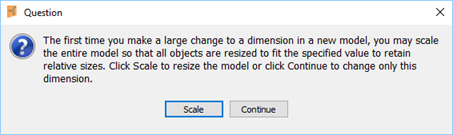From this message, click Scale. This will also change the height dimension so that its size remains relative to the width dimension.

7. Name the height dimension h and change its value to 4. The Parameter table should now look like the following.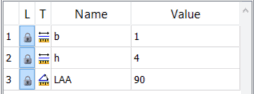8. Right-click in the sketch and select Zoom All from the shortcut menu to resize the display of the drawing. Reposition the dimensions so that your sketch looks similar to the following: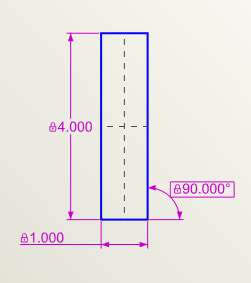9. Save the file as Beam.enb.

You have completed sketching a simple model of a beam. Next, we'll add equations to the model.

### Step 2: Add equations to the model

1. Add a new math group by selecting New Group... from the Group drop-down at the top of the Math view.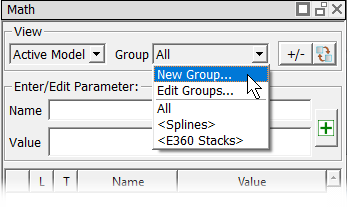2. Type Beam Equations as the name of the new group in the New Math Group dialog that opens, and click OK.

The new Beam Equations group is added to the Group drop-down list.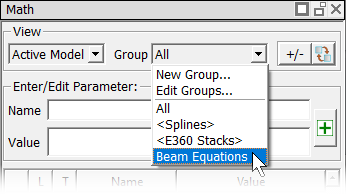3. To add the height and width dimensions to the Beam Equations group, extend-select (hold down the Ctrl key while clicking on the dimensions in either the sketch or parameter table) the h and b dimensions, and then select the box next to Beam Equations in the Properties Explorer.4. Select Beam Equations from the Group drop-down list to make the group active. Note that the Beam Equations group now contains the b and h dimensions.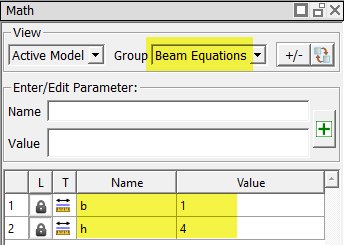5. In the equation table (the lower portion of the Math view), click the New Equation button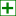to open the Equation Editor dialog, and type the basic beam equation for stress (S): S=M*c/Ix. (Note: You can copy each equation from this page and paste it into the Equation Editor.)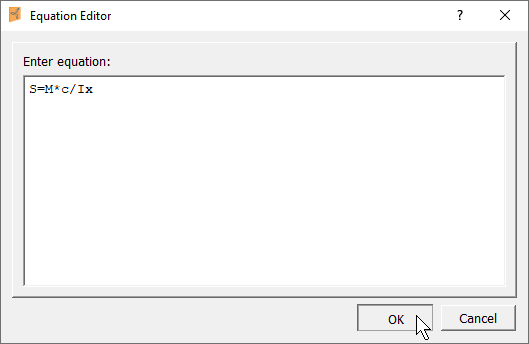6. Press Enter or click OK to add the equation. Note that the variables included in the equation have been added to the parameter table.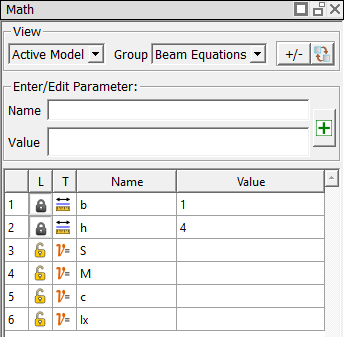7. Enter the following equations in the same way you did for the S equation:

• Area moment of Inertia about the x axis (Ix): Ix=b*h^3/12
• Value of distance to the neutral axis (c): c=h/2
• Moment (M): M=P*L/4

After you have entered all the equations, the Beam Equations group will contain the following entries.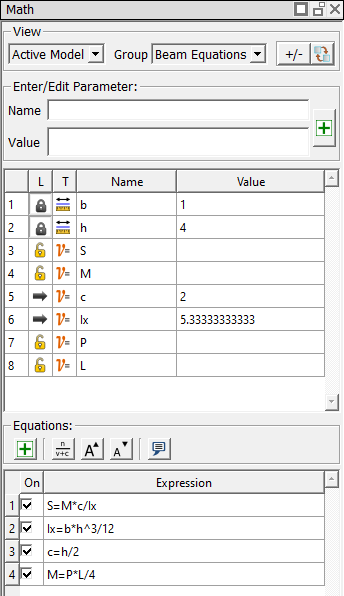8. In the parameter table, enter 1000 as the value for load (P) and 200 as the value for beam length (L). Based on the P and L values, Concept computes the moment (M) and stress (S) values.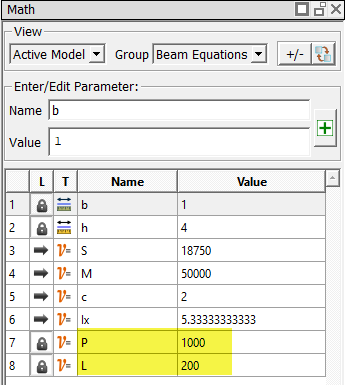9. Save the file.

# Tolerance analysis

This lesson illustrates how to analyze the variability of the computed parameters we created in Adding Equations to a Model.

1. Select Default Tolerances... from the Edit menu, and click the Dimensions tab to see the default settings for dimensions.
2. Change the default tolerance for linear dimensions to 0.01. Click OK to save your changes.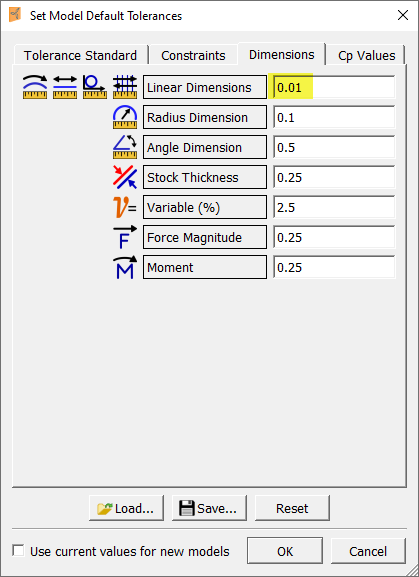3. Select the P variable from the parameter table.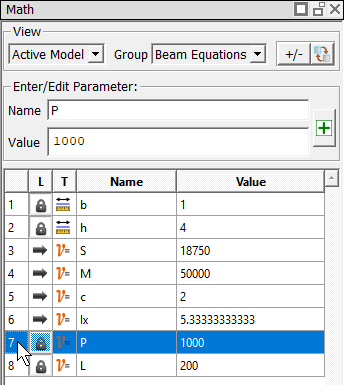4. In the Property Explorer’s Tolerance area, select +/- or Symmetric from the Type selection list, and specify a tolerance of +/- 50 as shown below.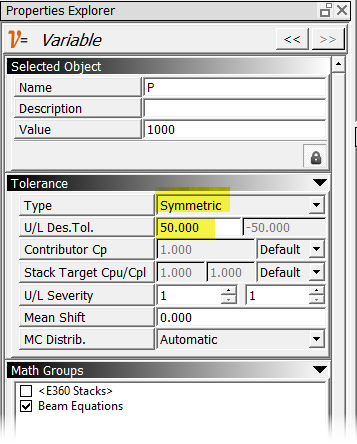5. In the same way you set the tolerance for the P variable, set the L variable's tolerance to +/- 0.1.
6. Select the S variable from the parameter table, and select Analyze Tolerance from the Analysis menu. Concept generates a tolerance report in the Modules view spreadsheet.

7. Click the maximize button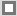in the upper right corner of the Modules view to maximize the view so you can see the entire tolerance analysis report. To see the Advanced Contributor section, scroll to the right of the report.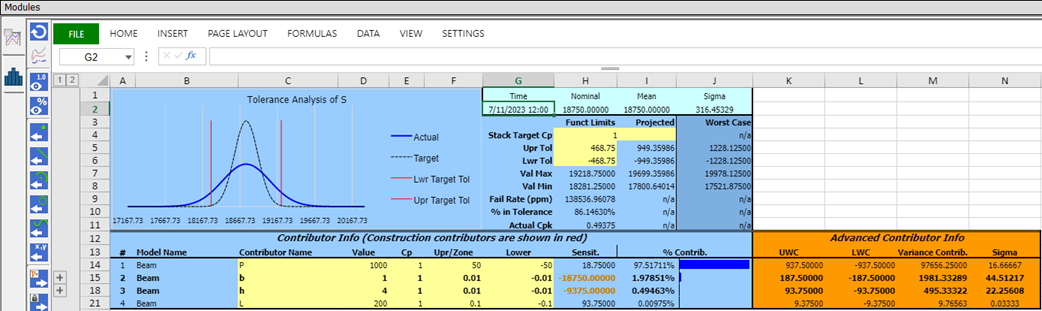8. To see the sub-contributors for the complex contributors b and h, click the expand button to the left of their rows.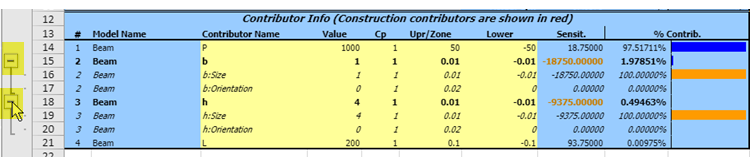9. Click the Restore buttonin the upper right corner of the maximized spreadsheet to return the Modules view to its normal docked size.
10. Save the file.

# Back-solving to derive a parameter value

In this lesson, you’ll learn how to back-solve from a computed variable to derive the value of a dimensional parameter. Continuing to work with the file we used in the previous lessons, Adding Equations to a Model and Tolerance Analysis, we’ll solve for the beam height that will produce a specific stress level. You will also learn how to add text to your sketch, which is particularly useful for sharing information about a model with other team members.

### Back-solve to drive the beam height

1. In the Math view’s parameter table, unlock the dimension by clicking on the lock symbol in the L (Lock Status) column.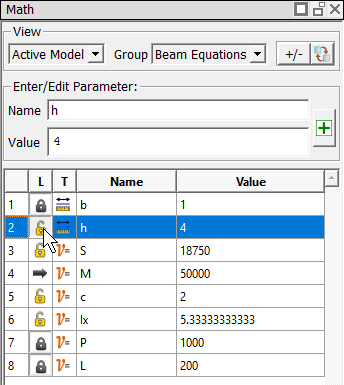2. Observe that the values of the Sc, and Ix variables also become unlocked. If we review the equations, we see that this occurs because these variables are interdependent. Entering a value for one of these variables (and thereby locking that variable) will drive the values of the other variables.
3. To drive the values with the stress (S) variable, type 10000 as the value for S, and press Enter to accept the change.

The status for S becomes Locked. The variables that are now driven by the value of S (hc, and Ix) are changed to Derived status, and their values are recalculated based on the value we entered for S.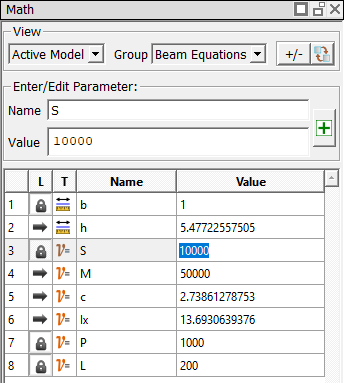Observe in the Sketch view that the height of the beam has changed in response to the new value of S, which now drives the beam height.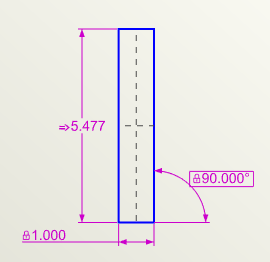### Add text to the sketch

In this part of the lesson, we'll add a note to the sketch to tell other team members that we used equations to find the height of the beam.

1. Select the Sketch Text tool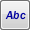from the Sketch view toolbar.
2. Select the line that has its height set by the h dimension.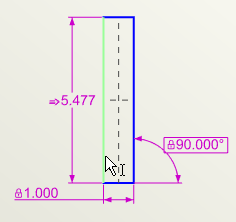3. In the Sketch Text dialog that appears, type the text shown in the following illustration, then click OK(Note: If you do not select an object when placing the Sketch Text, you will not have options to show a leader line or move the text with the object.)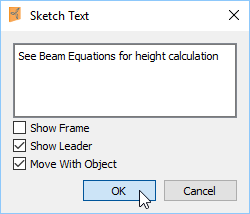4. If needed, zoom out or pan to see the text in its entirety.
5. Move the text as desired by clicking and dragging it using the Select tool. (You can return to the Select tool at any time by pressing Esc on your keyboard.)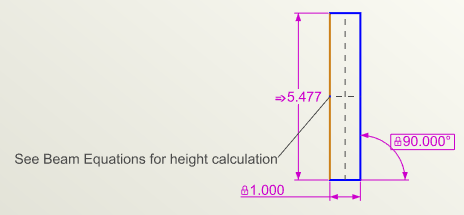6. Click on the text to see its properties in the Properties Explorer. To better format the text, add line breaks by pressing Enter to break the lines as shown in the following illustration.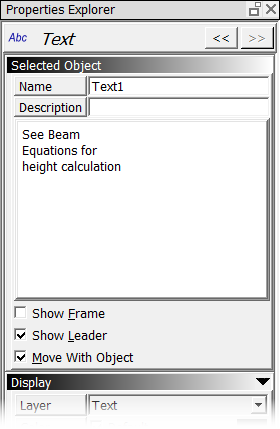The text now appears as shown below.Congratulations! You have successfully completed the Equations tutorial.

For extra practice, work through the attached labs that explore additional methods of computing beam stress.